## Prelude

Following Fasang and Liao (2014), we distinguish between sequence representation and summarization graphs. The latter aggregate and summarize the information stored in the sequence data without plotting actual observed sequences. Given the complexity of sequence data, these type of plots focus on one or two dimensions of information stored in sequence data (Brzinsky-Fay, 2014). Among the diverse members of the family of summarization graphs are sequence transitions plot, Kaplan-Meier survival curves, modal state plots, mean time plots, state distribution plots, and entropy plots (Fasang & Liao, 2014; Raab & Struffolino, 2022).

{ggseqplot} includes five summarization graphs:

• state distribution plots (ggseqdplot)
• entropy line plots (ggseqplot)
• modal state sequence plot (ggseqmsplot)
• mean time plot (ggseqmtplot)
• transition rate plots (ggseqtrplot)

Whereas summarization graphs aggregate the sequence data, representation plots always display actually observed sequences. In the most basic form of the traditional sequence index plot all observed sequences are displayed. In data sets with several hundred cases this kind of visualization, however, cannot be reasonably applied because of the issue of overplotting. In such a scenario individual sequences are partly plotted on top of each other and the resulting graph would be an inaccurate representation of the underlying sequence data. In response to this issue alternative representation plots which only render a subset of the sequences have been suggested. {ggseqplot} allows to render both the traditional sequence index plots and representation plots of subsets of sequences. Specifically, the library contains the following plot types:

• sequence index plot (ggseqiplot)
• sequence frequency plot (ggseqfplot)
• representative sequence plot (ggseqrplot)
• relative frequency sequence plot (ggseqrfplot)

For a more detailed discussion of sequence visualization I recommend the following articles/book chapters: Brzinsky-Fay (2014), Fasang and Liao (2014) and Chapter 2 of Raab & Struffolino (2022).

With the exception of the transition rate plot all of the plots listed above can be also produced with {TraMineR}. In total, those two libraries provide a much more comprehensive set of plots and often allow for more options than {ggseqplot}. Hence, if you are an experienced user base R’s plot (which is used to render the {TraMineR} plots), there is no real need to make yourself acquainted with {ggseqplot}.

{ggseqplot} was written because like many other R users I prefer {ggplot2} to base R’s plot environment for visualizing data. {TraMineR} (Gabadinho et al., 2011) was developed before {ggplot2} (Wickham, 2016) was as popular as it is today and back then many users were more familiar with coding base R plots. To date, however, many researchers and students are more accustomed to using {ggplot2} and prefer to draw on the related skills and experiences instead of learning how to refine base R plots just for the single purpose of visualizing sequence data.

This vignette outlines how sequence data generated with TraMineR::seqdef are reshaped to plot them as ggplot2-typed figures using {ggseqplot}. More specifically, it gives an overview of the general procedure and depicts which {TraMineR} and {ggplot2} functions are used to render the plots

The vignette further illustrates how the appearance of plots produced with {ggseqplot} can be changed using {ggplot2} functions and extensions.

## Setup example

We start by loading the required libraries and defining the sequence data to be plotted. We draw in the examples from the {TraMineR} for setting up the examples.

## ~~~~~~~~~~~~~~~~~~~~~~~~~~~~~~~~~~~~~~~~~~~~~~~~~~~~~~~
## ~~~~~~~~~~~~~~~~~~~~~~~~~~~~~~~~~~~~~~~~~~~~~~~~~~~~~~~

## Save package names as a vector of strings
pkgs <- c("colorspace", "ggplot2", "ggthemes", "hrbrthemes",
"patchwork", "purrr", "TraMineR")

## Install uninstalled packages
lapply(pkgs[!(pkgs %in% installed.packages())],
install.packages, repos = getOption("repos")["CRAN"])

lapply(pkgs, library, character.only = TRUE)

## Don't forget to load ggseqplot
library(ggseqplot)


## ~~~~~~~~~~~~~~~~~~~~~~~~~~~~~~~~~~~~~~~~~~~~~~~~~~~~~~~~~~~
## Creating state sequence objects from example data sets ----
## ~~~~~~~~~~~~~~~~~~~~~~~~~~~~~~~~~~~~~~~~~~~~~~~~~~~~~~~~~~~

## biofam data
data(biofam)

biofam.lab <- c("Parent", "Left", "Married", "Left+Marr",
"Child", "Left+Child", "Left+Marr+Child", "Divorced")

biofam.seq <- seqdef(biofam[501:600, ], 10:25, # we only use a subsample
labels = biofam.lab,
weights = biofam$wp00tbgs[501:600]) ## actcal data data(actcal) actcal.lab <- c("> 37 hours", "19-36 hours", "1-18 hours", "no work") actcal.seq <- seqdef(actcal,13:24, labels=actcal.lab) ## ex1 data data(ex1) ex1.seq <- seqdef(ex1, 1:13, weights=ex1$weights)

Note that the default figure size in this document is specified as: fig.width=8, fig.height=4.94

## Technicalities

In general, all {ggseqplot} functions operate in the similar way: they extract the data to be plotted using a state sequence object generated with TraMineR::seqdef as a staring point. The functions either simply use (a subset of) the sequence data stored in this object or call other {TraMineR} functions such as TraMineR::seqstatd to obtain the information to be plotted. Under the hood {ggseqplot} reshapes those data to visualize them using {ggplot2} functions. Usually this means that the data have to be reshaped into a long (tidy) format.

The following example illustrates the procedure for the case of a state distribution plot. The cross-sectional state distributions across the positions of the sequence data can be obtained by:

seqstatd(actcal.seq)
#>   [State frequencies]
#>   jan00 feb00 mar00 apr00 may00 jun00 jul00 aug00 sep00 oct00 nov00 dec00
#> A 0.421 0.420 0.421 0.417 0.419  0.42  0.42  0.42  0.42 0.418 0.414 0.415
#> B 0.162 0.162 0.162 0.162 0.162  0.16  0.16  0.17  0.16 0.169 0.170 0.171
#> C 0.098 0.098 0.098 0.097 0.097  0.10  0.10  0.10  0.10 0.096 0.096 0.098
#> D 0.320 0.321 0.320 0.324 0.322  0.32  0.32  0.31  0.31 0.318 0.320 0.316
#>
#>    [Valid states]
#>   jan00 feb00 mar00 apr00 may00 jun00 jul00 aug00 sep00 oct00 nov00 dec00
#> N  2000  2000  2000  2000  2000  2000  2000  2000  2000  2000  2000  2000
#>
#>    [Entropy index]
#>   jan00 feb00 mar00 apr00 may00 jun00 jul00 aug00 sep00 oct00 nov00 dec00
#> H   0.9   0.9   0.9   0.9   0.9   0.9   0.9  0.91  0.91   0.9  0.91  0.91

When calling ggseqdplot these distributional data are reshaped into a long data set in which every row stores the (weighted) relative frequency of a given state at a given position along the sequence. The example data actcal.seq contain sequences of length 12 with an alphabet comprising 4 states. The reshaped data serving as source for the {ggplot2} call thus contain $$12\times4=48$$ rows. If a group vector is specified, the respective data will comprise 48 rows for each group. The data set produced by ggseqdplot can be accessed if the function’s output is assigned to an object. The resulting list object stores the data as its first element (named data).

dplot <- ggseqdplot(actcal.seq)
dplot$data #> # A tibble: 48 × 6 #> group state k x value grouplab #> <fct> <fct> <fct> <fct> <dbl> <fct> #> 1 1 > 37 hours jan00 1 0.421 Rel. Freq. (n=2000) #> 2 1 > 37 hours feb00 2 0.420 Rel. Freq. (n=2000) #> 3 1 > 37 hours mar00 3 0.422 Rel. Freq. (n=2000) #> 4 1 > 37 hours apr00 4 0.418 Rel. Freq. (n=2000) #> 5 1 > 37 hours may00 5 0.420 Rel. Freq. (n=2000) #> 6 1 > 37 hours jun00 6 0.42 Rel. Freq. (n=2000) #> 7 1 > 37 hours jul00 7 0.422 Rel. Freq. (n=2000) #> 8 1 > 37 hours aug00 8 0.420 Rel. Freq. (n=2000) #> 9 1 > 37 hours sep00 9 0.418 Rel. Freq. (n=2000) #> 10 1 > 37 hours oct00 10 0.418 Rel. Freq. (n=2000) #> # ℹ 38 more rows Once the data are in the right shape {ggseqplot} functions produce graphs using {ggplot2} functions. In the case of the state distribution plot, for instance, ggseqdplot renders stacked bar charts for each sequence position using ggplot2::geom_bar. The following table gives an overview of the most important internal function calls used to render different plot types with {ggseqplot} ggseqplot function TraMineR function ggplot2 geoms and extensions ggseqdplot TraMineR::seqstatd ggplot2::geom_bar optional: geom_line ggseqeplot TraMineR::seqstatd ggplot2::geom_line ggseqmsplot TraMineR::seqmodst ggplot2::geom_bar ggseqmtplot TraMineR::seqmeant ggplot2::geom_bar ggseqtrplot TraMineR::seqtrate ggplot2::geom_tile ggseqiplot TraMineR::seqformat ggplot2::geom_rect ggseqfplot TraMineR::seqtab ggplot2::geom_rect {ggh4x} (for the axis labeling if group has been specified) ggseqrplot TraMineR::seqrep ggplot2::geom_rect ggrepel::geom_text_repel {ggtext} (for optional colored axis labels) {patchwork} (to combine plots) ggseqrfplot TraMineR::seqrfplot ggplot2::geom_rect ggplot2::geom_boxplot {patchwork} (to combine plots) The appearance of most of the plots generated with {ggseqplot} can be adjusted just like every other ggplot (e.g., by changing the theme or the scale using + and the respective functions). Representative sequence plots and relative frequency sequence plots, however, behave differently because they are composed of multiple plots which are arranged by the {patchwork} library. The following section illustrates how the appearance of the plots can be changed ## Changing the appearance of plots ### The default case As mentioned above, most plots rendered by {ggseqplot} are of class c("gg", "ggplot") and can be adjusted just like other plots rendered with {ggplot2} #### Example 1: State distribution plot In our first example we illustrate this for state distribution plot. We start with the most basic version of the plot visualizing the state distributions of actcal.seq without changing any of the defaults. # ggseqplot::ggseqdplot ggseqdplot(actcal.seq)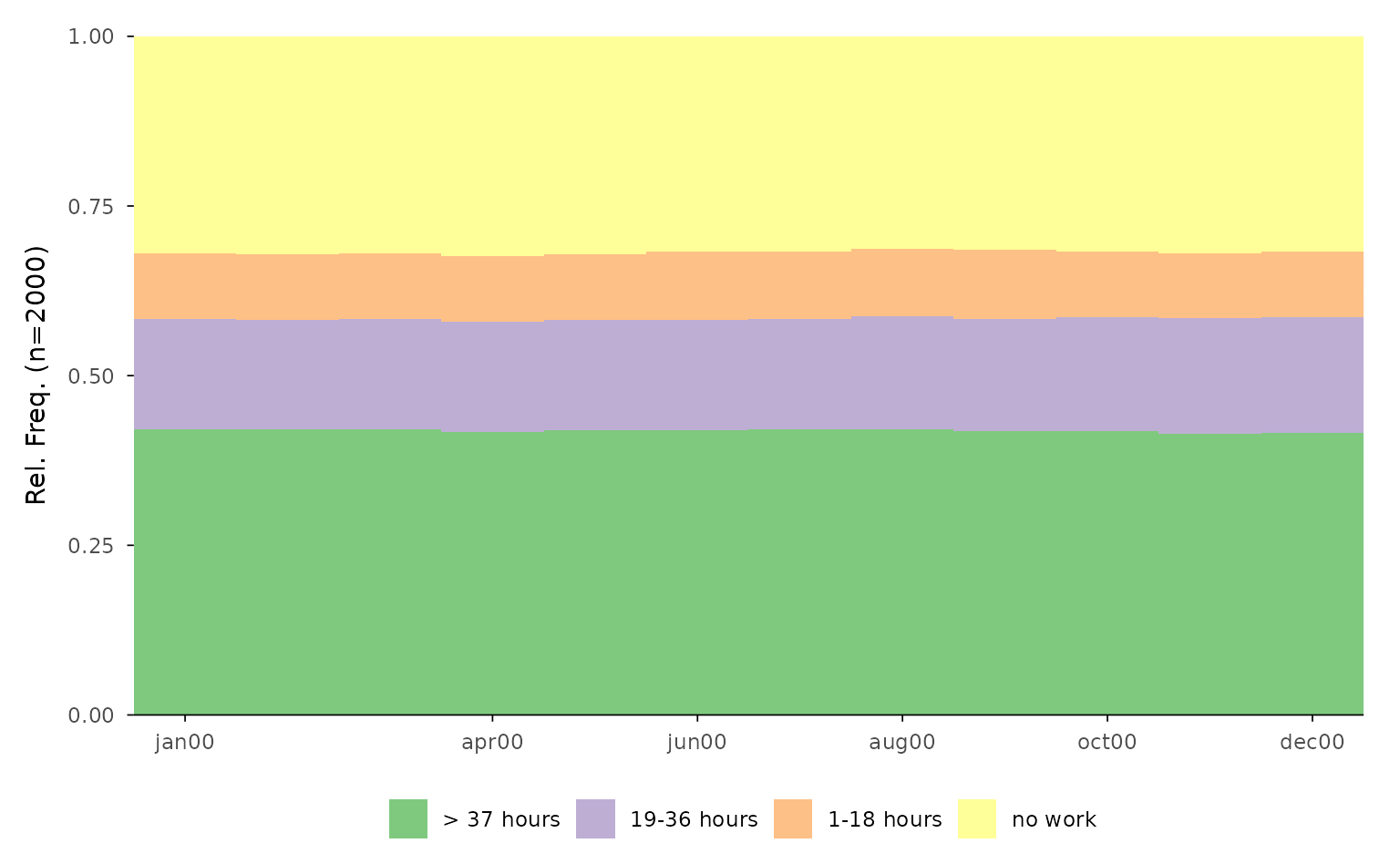We proceed by illustrating how {ggplot2} functions & extensions can be used to refine the default outcome. Just like every other {ggplot2} figure the appearance of plots generated with {ggseqplot} functions can be dramatically changed with a few adjustments: ggseqdplot(actcal.seq) + scale_fill_discrete_sequential("heat") + scale_x_discrete(labels = month.abb) + labs(title = "State distribution plot", x = "Month") + guides(fill=guide_legend(title="Alphabet")) + theme_ipsum(base_family = "") + # ensures that this works on different OS theme(plot.title = element_text(size = 30, margin=margin(0,0,20,0)), plot.title.position = "plot")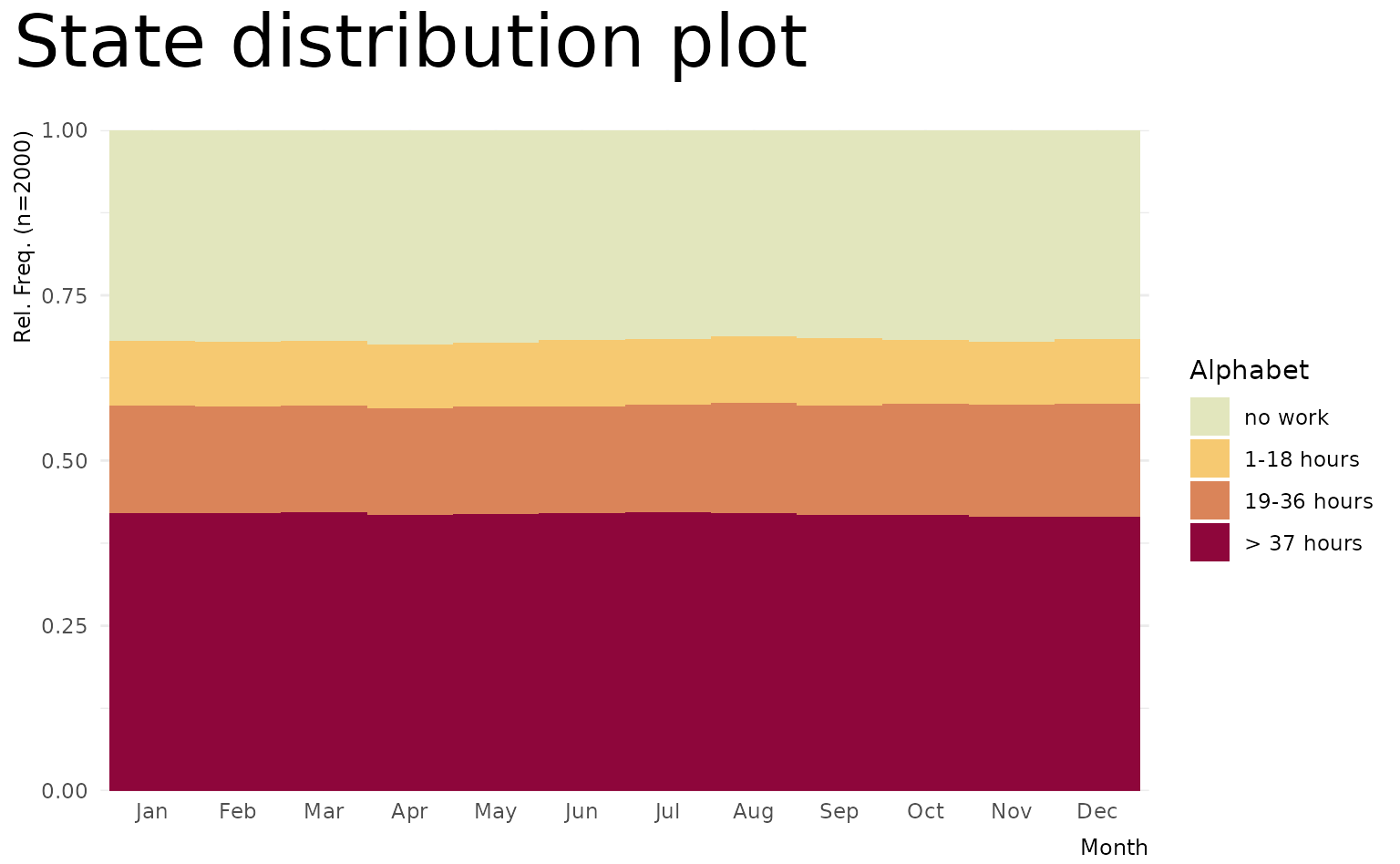In the following example we again illustrate a few {ggplot2} functions & extensions by composing a figure comprising two plots produced with ggseqdplot. Both visualize the same data but only the first plot considers weights. In addition to state distributions the plots display the accompanying entropies as line plot (geom_line). Finally, the plots are brought together using the {patchwork} library (Pedersen, 2020). # Save plot using weights p1 <- ggseqdplot(ex1.seq, with.entropy = TRUE) + ggtitle("Weighted data") # Save same plot without using weights p2 <- ggseqdplot(ex1.seq, with.entropy = TRUE, weighted = FALSE) + ggtitle("Unweighted data") # Arrange and refine plots using patchwork p1 + p2 + plot_layout(guides = "collect") & scale_fill_manual(values= canva_palettes$Fun and tropical[1:4]) &
theme_ipsum(base_family = "") &
theme(plot.title = element_text(size = 20,
hjust = 0.5),
legend.position = "bottom",
legend.title = element_blank())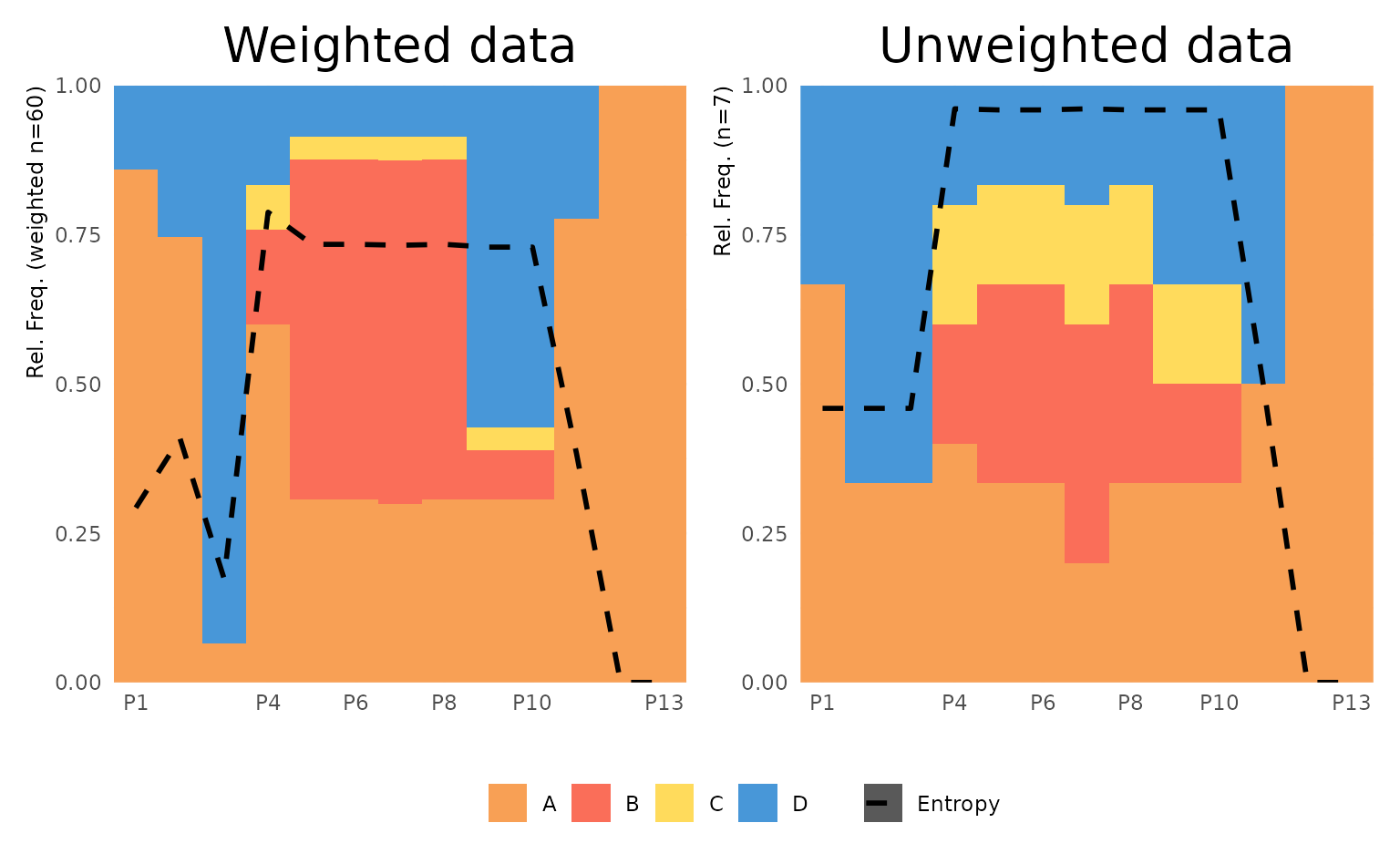#### Example 2: Transition rate plot

The second set of examples illustrates how to refine a figure of combined transition rate plots. ggseqtrplot calls TraMineR::seqtrate to obtain the transition rates between the states of the alphabet. TraMineR::seqtrate stores these rates in a symmetrical matrix which internally is reshaped into a long format with one row for every combination of states (i.e., the squared size of the sequence alphabet) by ggseqdplot. The reshaped data are the input for a {ggplot2} call using geom_tile.

We start with a simple example that only takes the sequence data and the group argument as inputs. The output is a faceted plot visualizing two transition rate matrices of DSS sequence data.

ggseqtrplot(actcal.seq,
group = actcal$sex) #> [>] computing transition probabilities for states A/B/C/D ... #> [>] computing transition probabilities for states A/B/C/D ...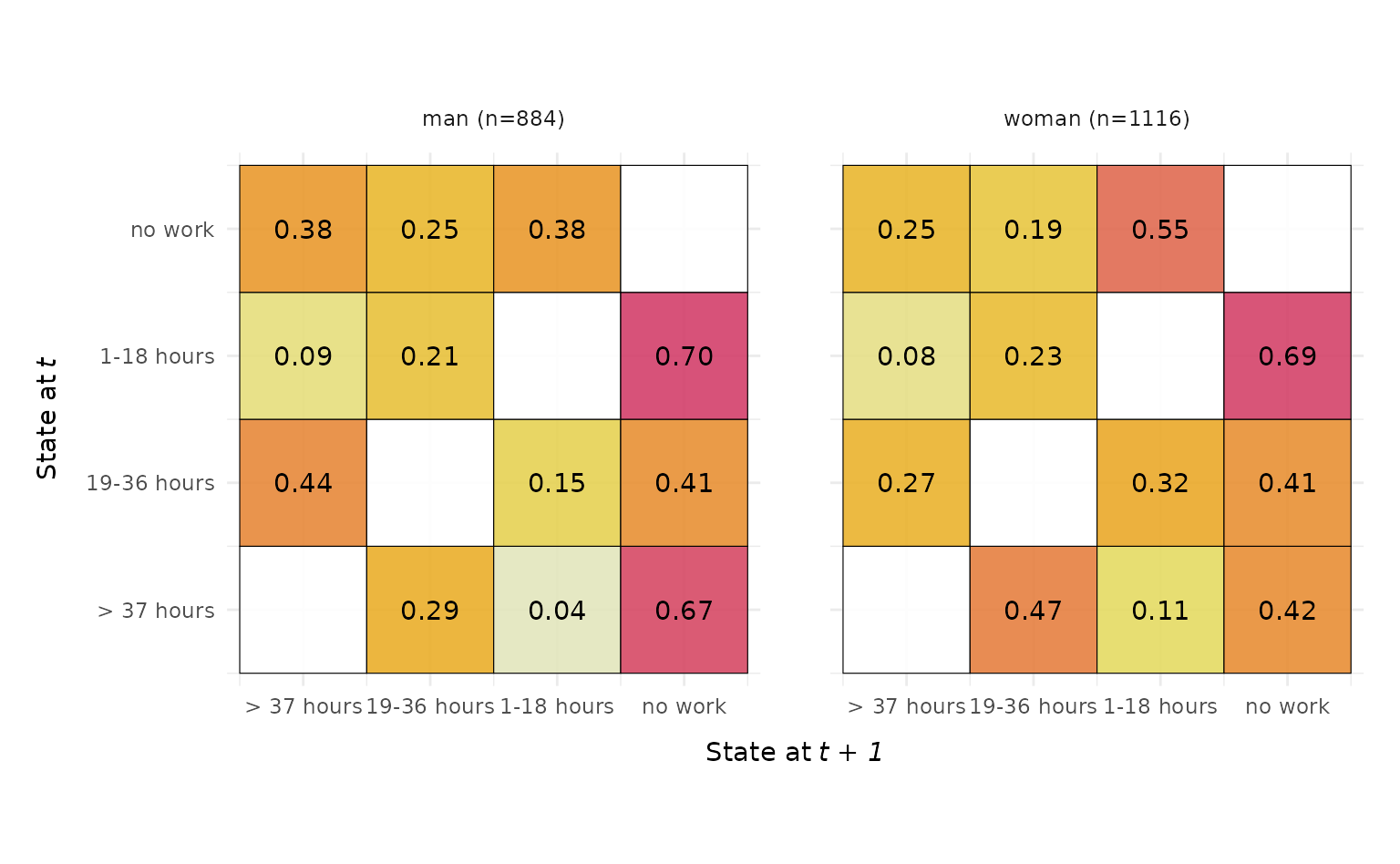In the second example we specify additional arguments and utilize once again the {patchwork} library to compose a figure that compares the transition matrices of sequence stored in the STS and the DSS format. We use x_n.dodge = 2 to prevent overlapping of the state labels of the x-axis, slightly reduce the labels size of the value labels displayed within the tiles, and use dss = FALSE to compute and display the transition rates of the STS sequences.  p1 <- ggseqtrplot(biofam.seq, dss = FALSE, x_n.dodge = 2, labsize = 3) + ggtitle("STS Sequences") + theme(plot.margin = unit(c(5,10,5,5), "points")) #> [>] computing transition probabilities for states 0/1/2/3/4/5/6/7 ... p2 <- ggseqtrplot(biofam.seq, x_n.dodge = 2, labsize = 3) + ggtitle("DSS Sequences") + theme(plot.margin = unit(c(5,5,5,10), "points")) #> [>] computing transition probabilities for states 0/1/2/3/4/5/6/7 ... p1 + p2 & theme(plot.title = element_text(size = 20, hjust = 0.5))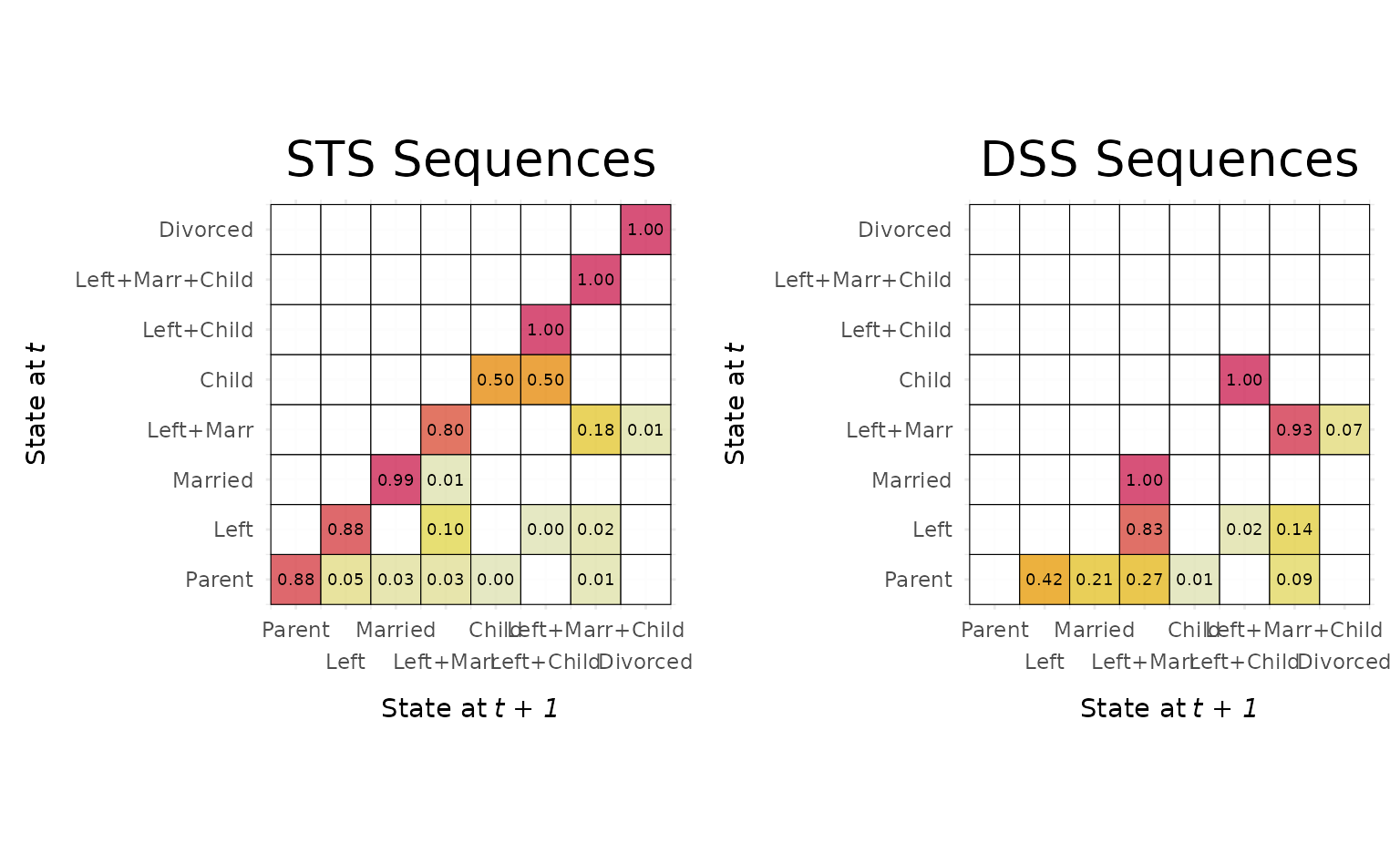Other than the grouped version of the plot this composed figure contains the y-axis title and labels twice. This can be changed with small adjustments of the corresponding theme arguments.  p2 <- p2 + theme(axis.text.y = element_blank(), axis.title.y = element_blank()) p1 + p2 & theme(plot.title = element_text(size = 20, hjust = 0.5))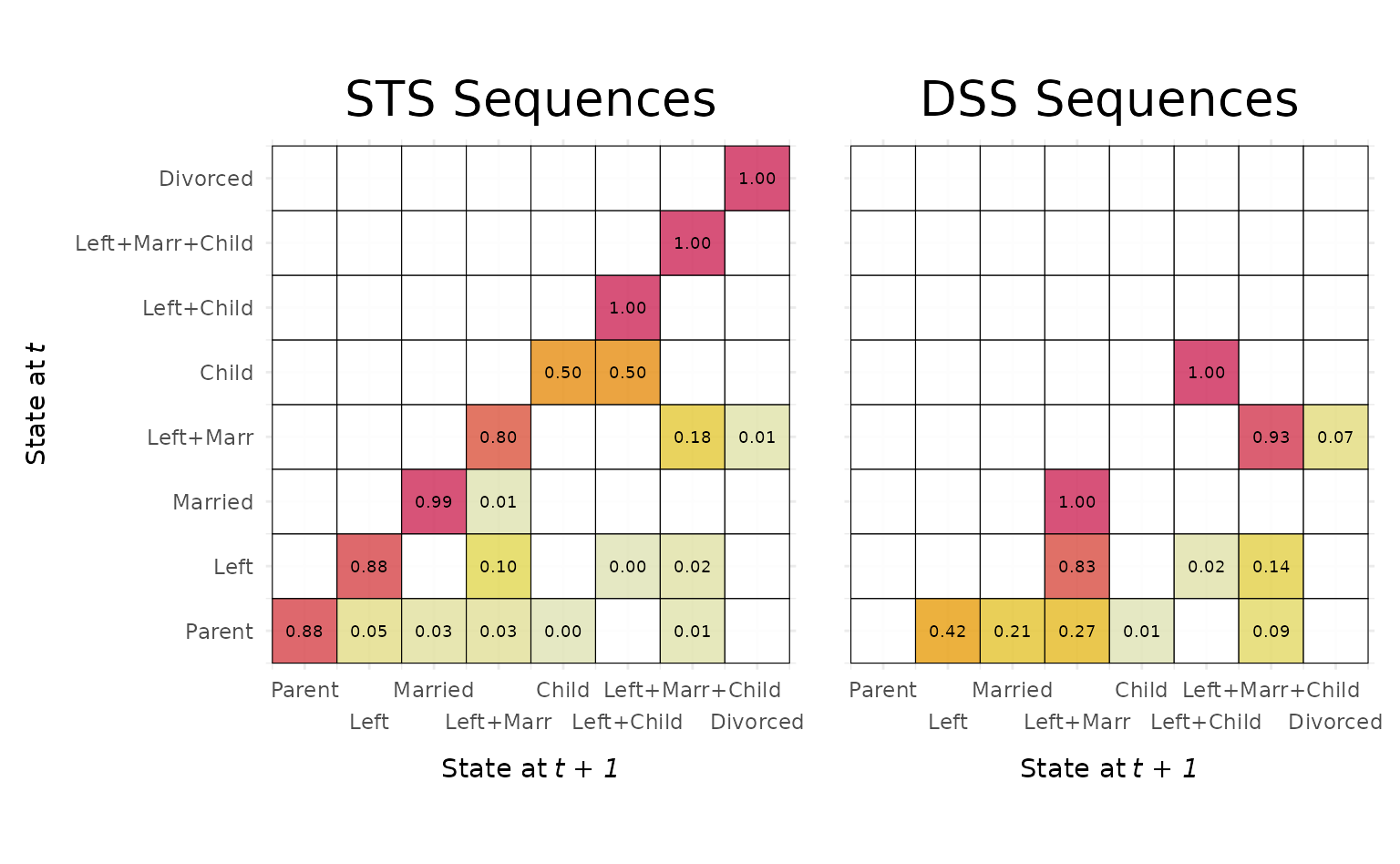#### Example 3: Flipping coordinates We conclude this section by illustrating that it is also possible to flip the coordinates of the plots rendered by {ggseqplot}, a procedure that is widely used in the {ggplot2} universe (although the coordinates could also be swapped in the aes(x, y, ...) specification). In the example below we illustrate the procedure for a mean time plot and a sequence index plot. We always present both, the default plot and the flipped version: ## default plot ggseqmtplot(actcal.seq, no.n = TRUE, error.bar = "SE")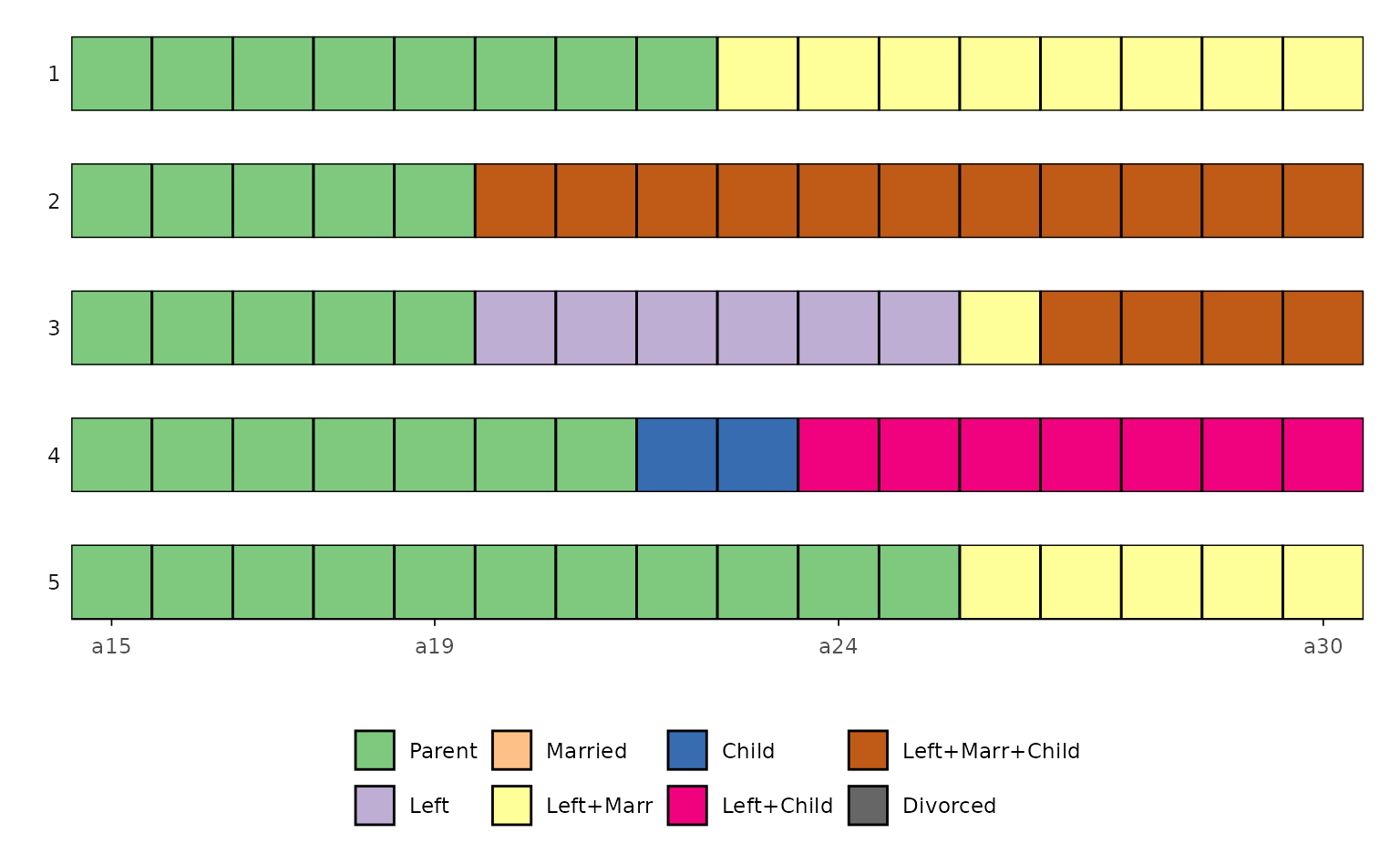## flipped version ggseqmtplot(actcal.seq, no.n = TRUE, error.bar = "SE") + coord_flip() + theme(axis.text.y=element_blank(), axis.ticks.y = element_blank(), panel.grid.major.y = element_blank(), legend.position = "top")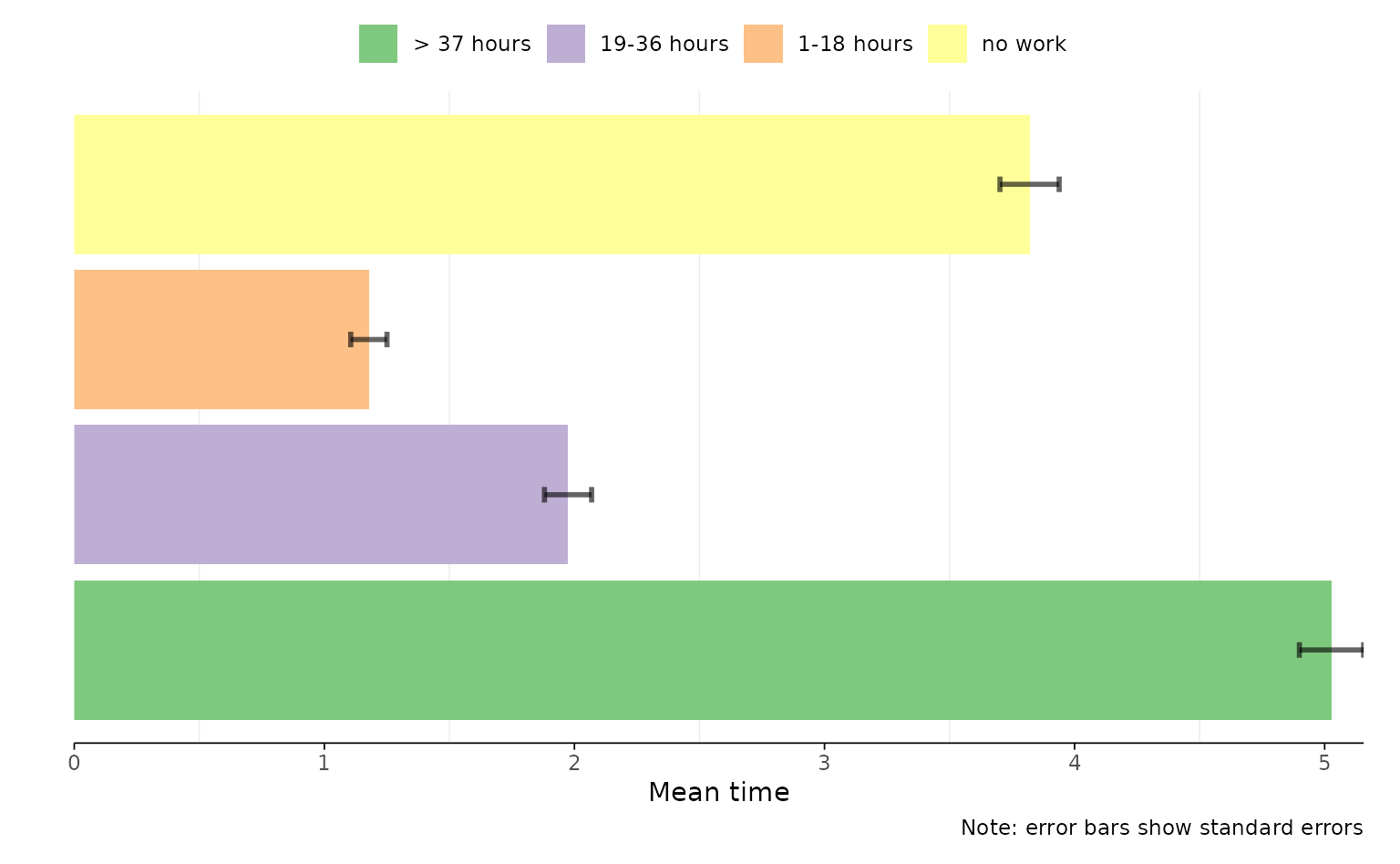While in the example above the flipped plot might be in greater accordance to most people’s aesthetic preferences, flipping the coordinates in the case of sequence index plots might be a more of an opinionated design choice. Most scholars prefer to display time on the horizontal axis. However, if you favor time to run from the bottom to the top (like in Piccarreta and Lior (2010)) instead of left to right, your preferences can be easily met. ## default plot ggseqiplot(actcal.seq, sortv = "from.end") + scale_x_discrete(labels = month.abb) #> Scale for x is already present. #> Adding another scale for x, which will replace the existing scale.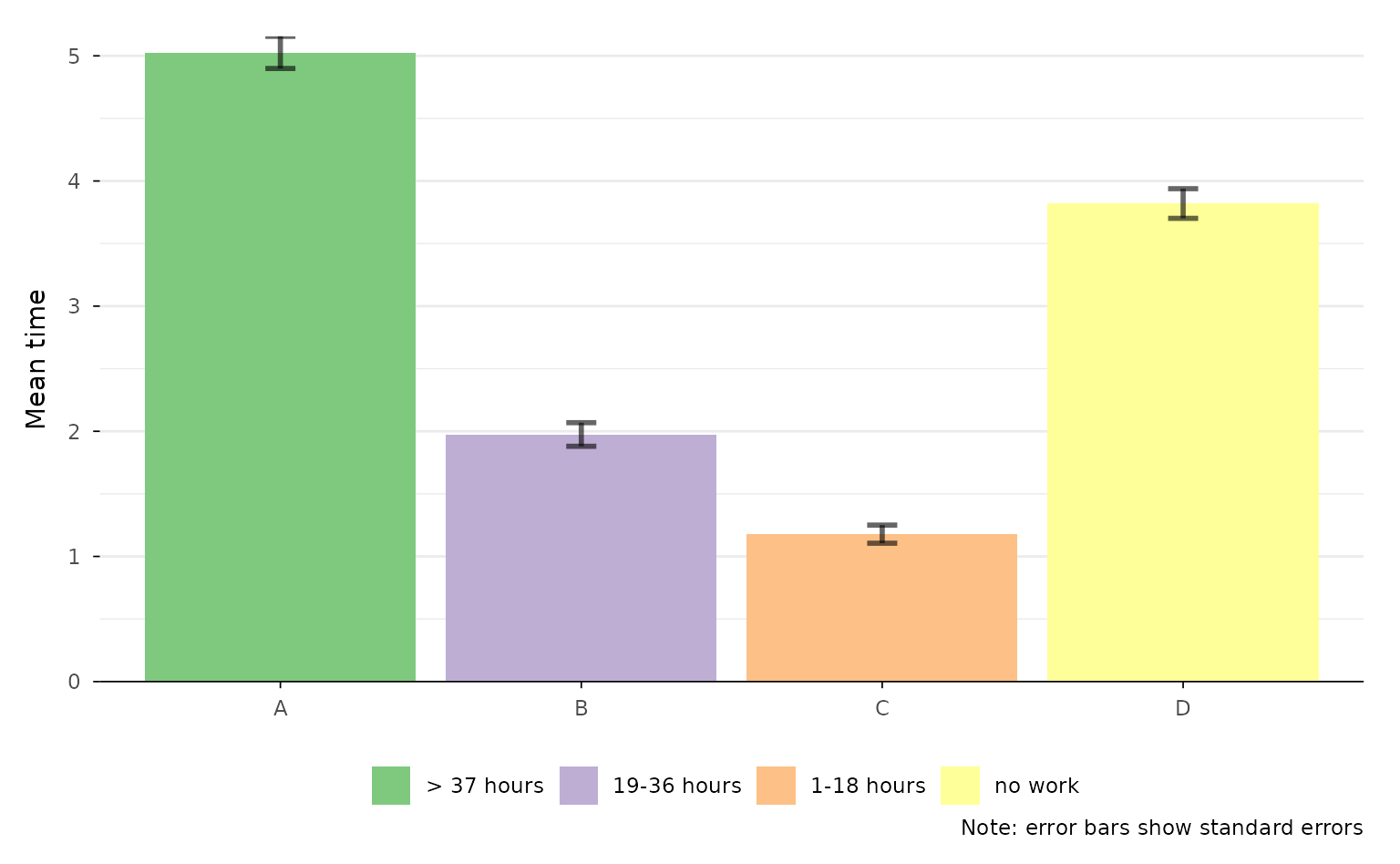## flipped version ggseqiplot(actcal.seq, sortv = "from.end") + scale_x_discrete(labels = month.abb) + coord_flip() #> Scale for x is already present. #> Adding another scale for x, which will replace the existing scale.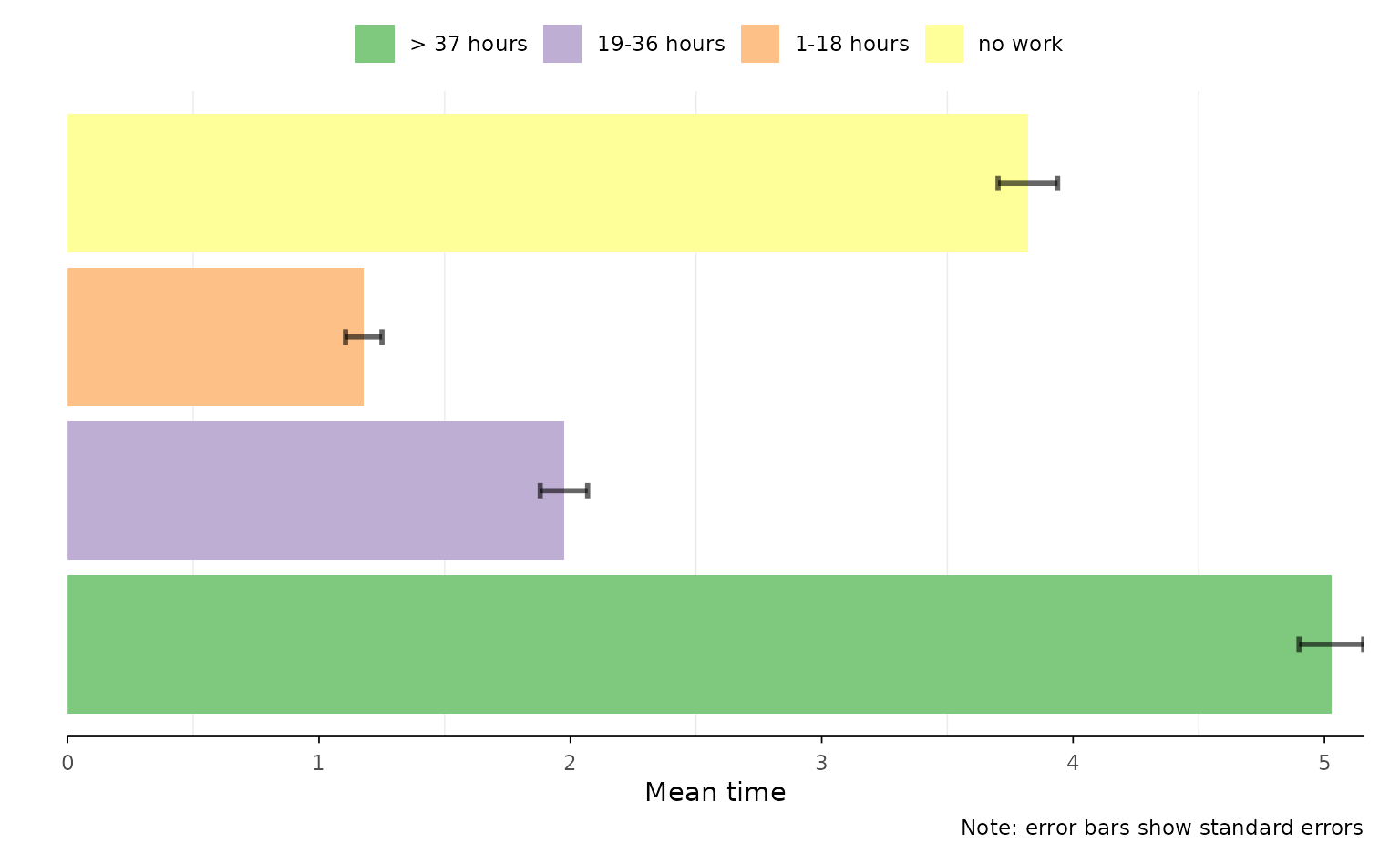### The special case of combined plots Two types of plots differ from the other {ggseqplot} functions because they are composed by two subplots which are arranged to a joint figure with the {patchwork} library. The output of those functions cannot be changed in the same was as for the other functions. For details on the {patchwork} library we recommend the package’s website. #### Example 4: Annotation and themes Some of the adjustments of a combined {patchwork} plot are pretty similar to the default {ggplot2} procedure. In the example below we change the theme and add a title to the plot. Note that the corresponding functions are not added by + but with & instead. ## compute dissimilarity matrix required for plot diss <- seqdist(biofam.seq, method = "LCS") #> [>] 100 sequences with 8 distinct states #> [>] creating a 'sm' with a substitution cost of 2 #> [>] creating 8x8 substitution-cost matrix using 2 as constant value #> [>] 76 distinct sequences #> [>] min/max sequence lengths: 16/16 #> [>] computing distances using the LCS metric #> [>] elapsed time: 0.02 secs ## Relative Frequency Sequence Plot ## default version ggseqrfplot(biofam.seq, diss = diss, k = 12) #> [>] Using k=12 frequency groups with grp.meth='prop' #> [>] Pseudo/medoid-based-R2: 0.367766 #> [>] Pseudo/medoid-based-F statistic: 5.268049, p-value: 1.655783e-06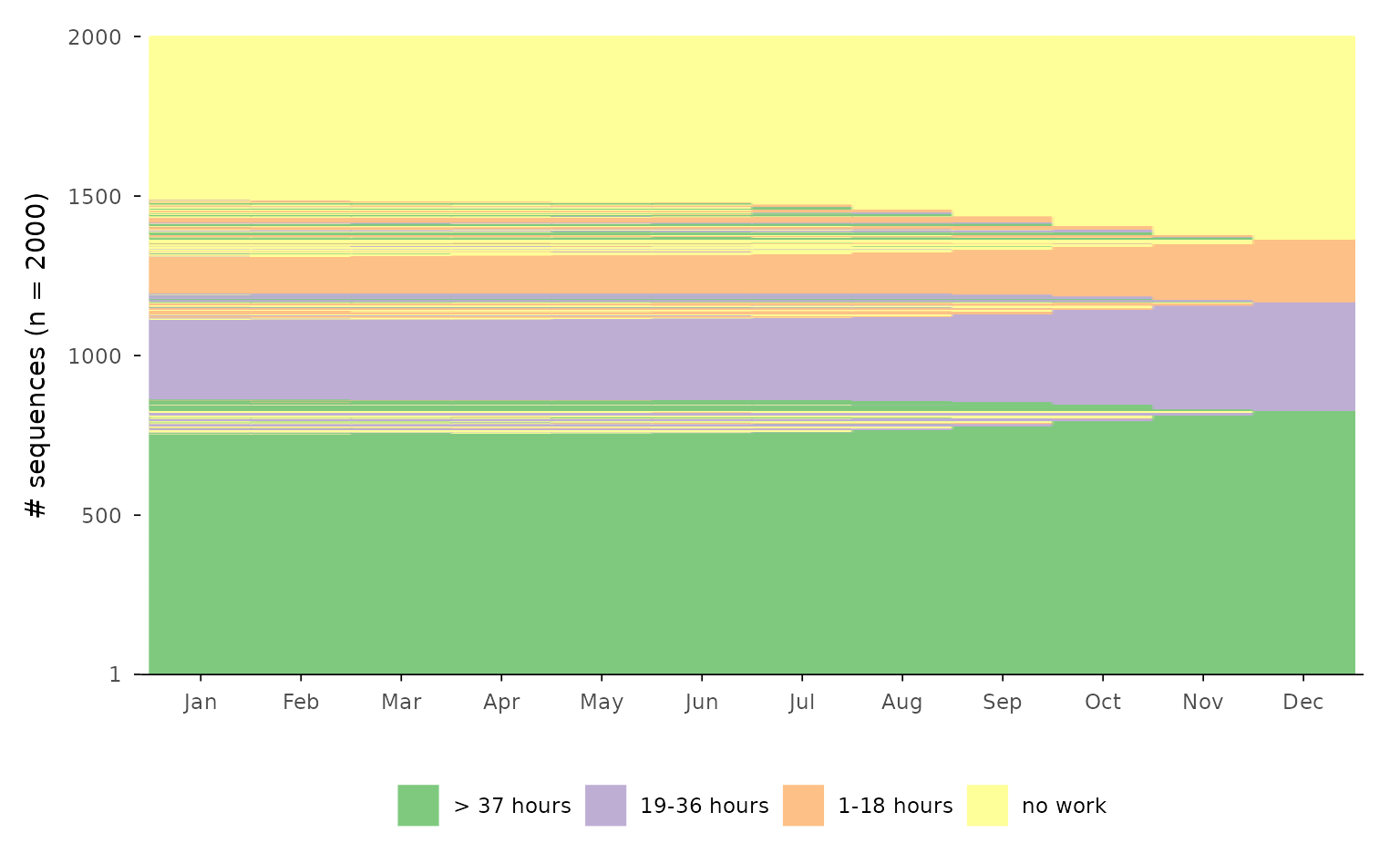## adjusted version ggseqrfplot(biofam.seq, diss = diss, k = 12) & theme_ipsum(base_family = "") & theme(legend.position = "bottom", legend.title = element_blank(), plot.title = element_text(size = 12)) & plot_annotation(title = "Relative Frequency Sequence Plot") #> [>] Using k=12 frequency groups with grp.meth='prop' #> [>] Pseudo/medoid-based-R2: 0.367766 #> [>] Pseudo/medoid-based-F statistic: 5.268049, p-value: 1.655783e-06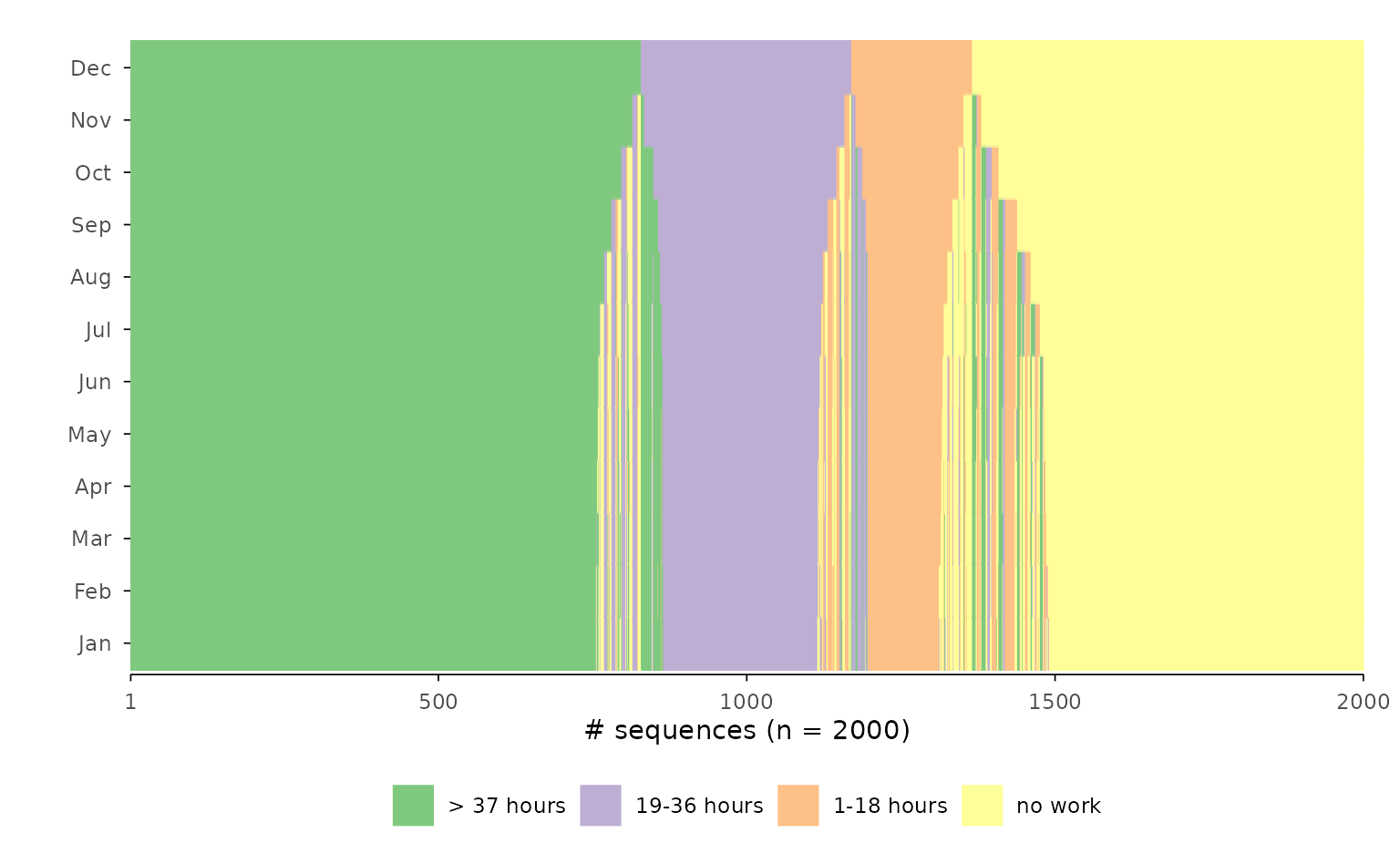If you want to manipulate the appearance of a specific plot, however, your default code might not work. If you want to change the labels of the index plot, for instance, the following code will not produce the desired result, because scale_x_discrete will change the appearance of the boxplot, i.e. the last plot used when composing the plot with {patchwork}. ggseqrfplot(biofam.seq, diss = diss, k = 12) + scale_x_discrete(labels = 15:30) #> [>] Using k=12 frequency groups with grp.meth='prop' #> [>] Pseudo/medoid-based-R2: 0.367766 #> [>] Pseudo/medoid-based-F statistic: 5.268049, p-value: 1.655783e-06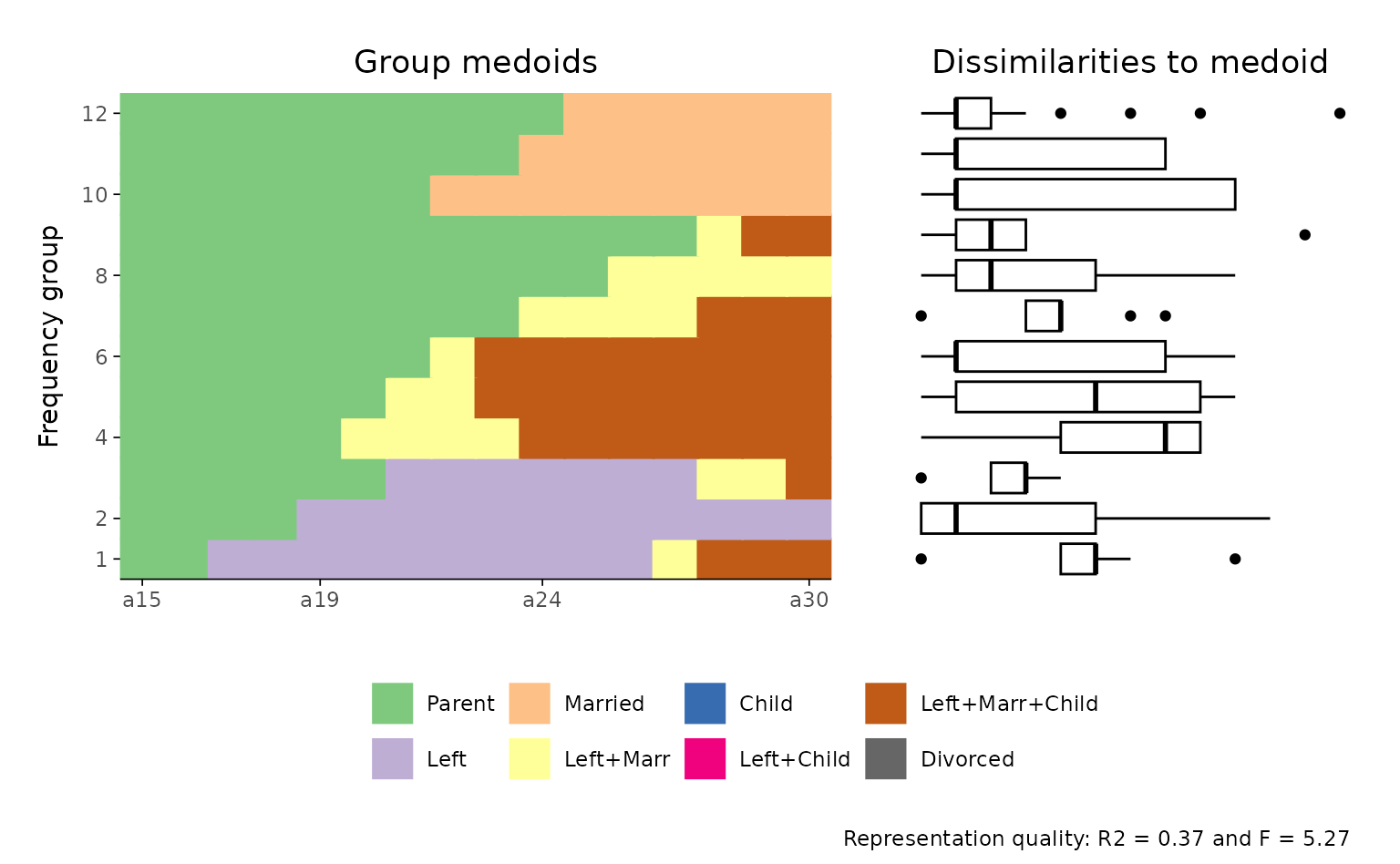The appearance of the subplots, however, can be changed once you save the composite plot and then adjust its components. ## save & view original plot p <- ggseqrfplot(biofam.seq, diss = diss, k = 12) #> [>] Using k=12 frequency groups with grp.meth='prop' #> [>] Pseudo/medoid-based-R2: 0.367766 #> [>] Pseudo/medoid-based-F statistic: 5.268049, p-value: 1.655783e-06 p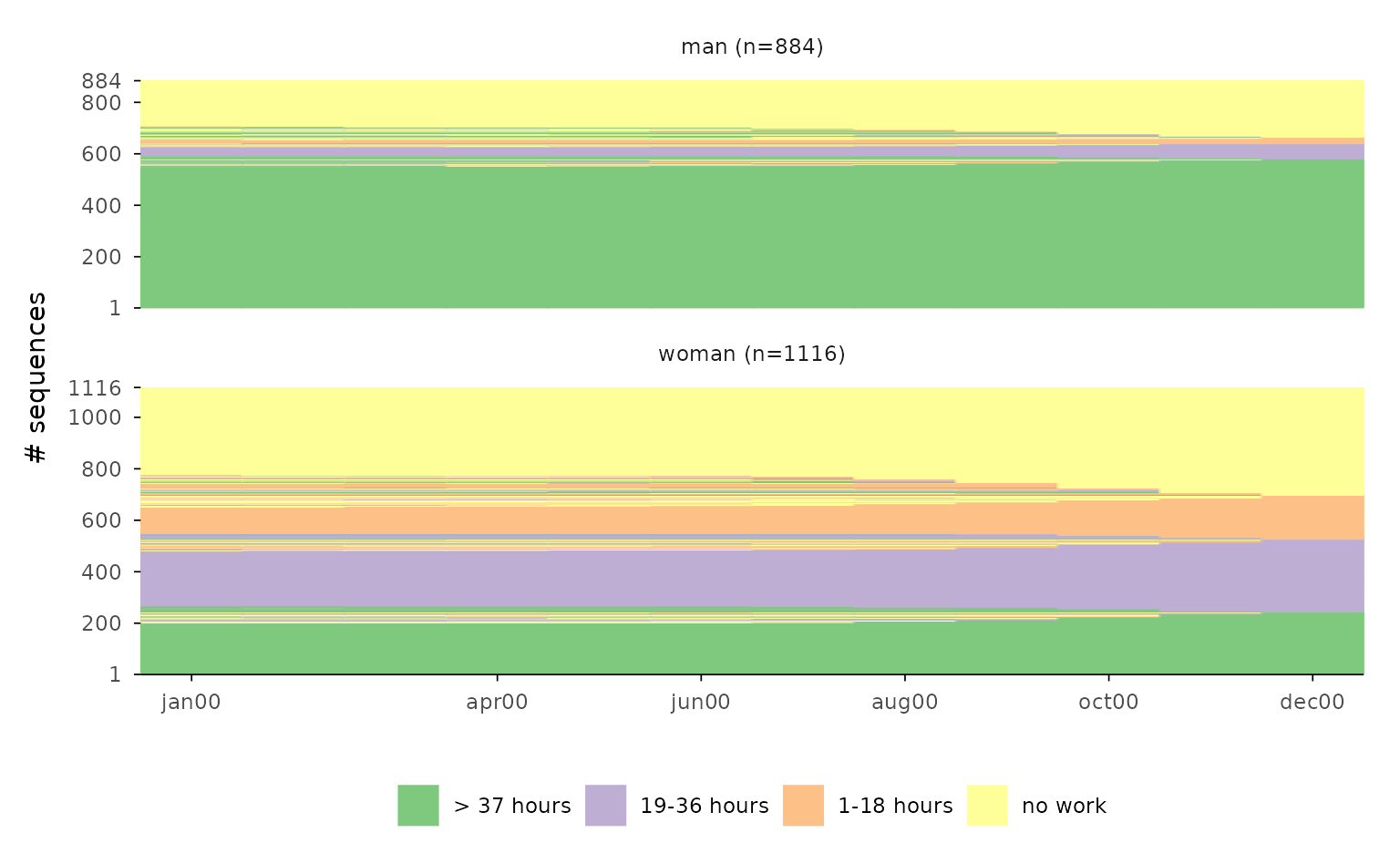## change appearance of sub-plots ## first component: index plot p[] <- p[] + scale_x_discrete(labels = 15:30) #> Scale for x is already present. #> Adding another scale for x, which will replace the existing scale. ## second component: boxplot p[] <- p[] + labs(title = "Changed title") ## adjusted plot p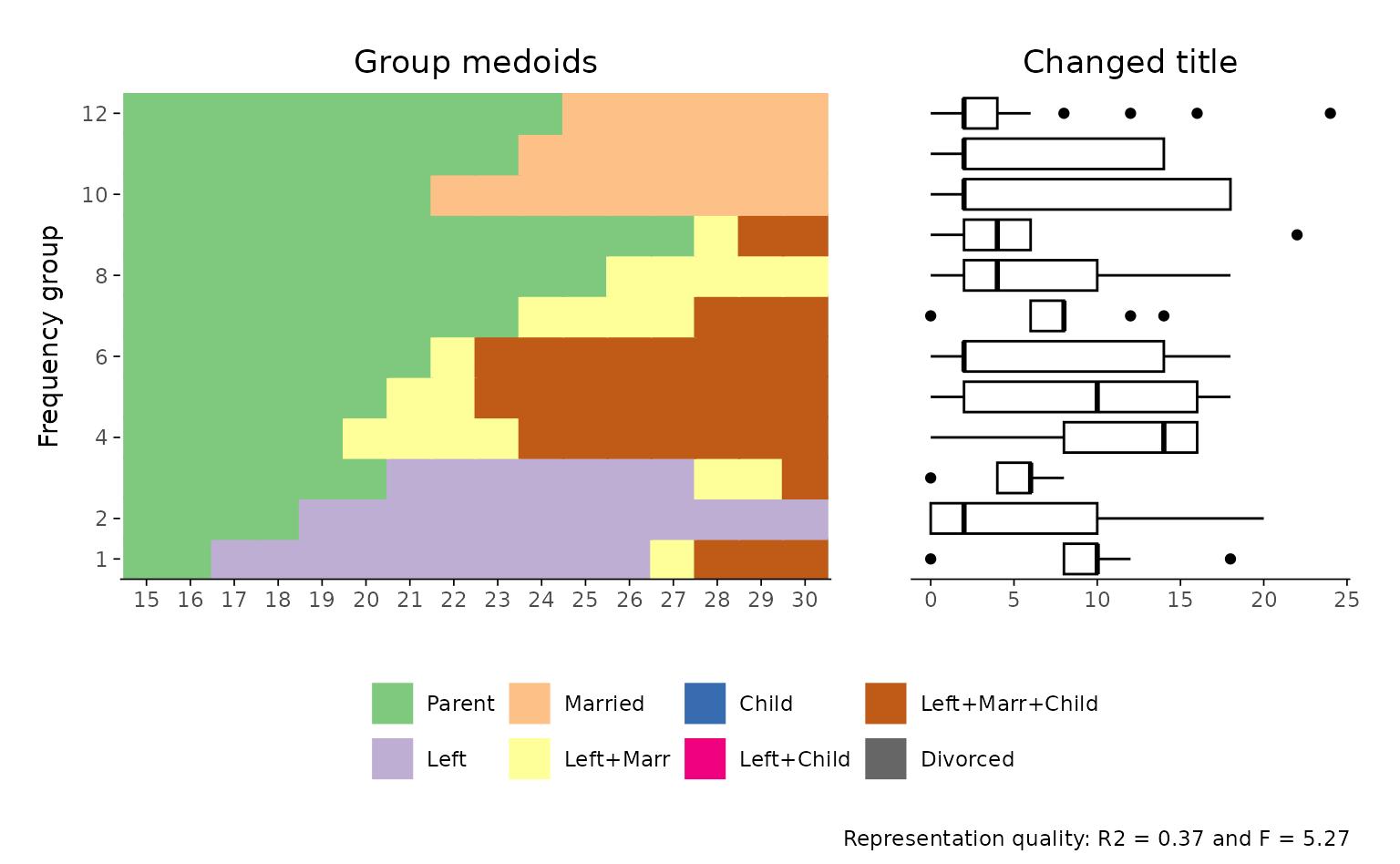#### Example 5: The complex case of grouped rplots Note that things become a little bit more complex in the case of a grouped representative sequence plot. In such a plot each group contributes two subplots. One providing information on the “quality” of the representative sequences, and another one containing the corresponding index plots. If we want to change the x-axis labels of the following plot, we have to extract and change the index plots which appear in the second row of the combined plot. The plots are arranged by row. Hence the index plots are the subplots 3 and 4 ## Compute a pairwise dissimilarity matrix diss <- seqdist(actcal.seq, method = "LCS") #> [>] 2000 sequences with 4 distinct states #> [>] creating a 'sm' with a substitution cost of 2 #> [>] creating 4x4 substitution-cost matrix using 2 as constant value #> [>] 186 distinct sequences #> [>] min/max sequence lengths: 12/12 #> [>] computing distances using the LCS metric #> [>] elapsed time: 0.27 secs ## original plot p <- ggseqrplot(actcal.seq, diss = diss, nrep = 3, group = actcal$sex)
#>  [>] number of objects (sum of weights): 884
#>  [>] max. distance: 24
#>  [>] 3 representative(s) selected
#>  [>] 80 distinct sequence(s)
#>  [>] number of objects (sum of weights): 1116
#>  [>] max. distance: 24
#>  [>] 3 representative(s) selected
#>  [>] 144 distinct sequence(s)
p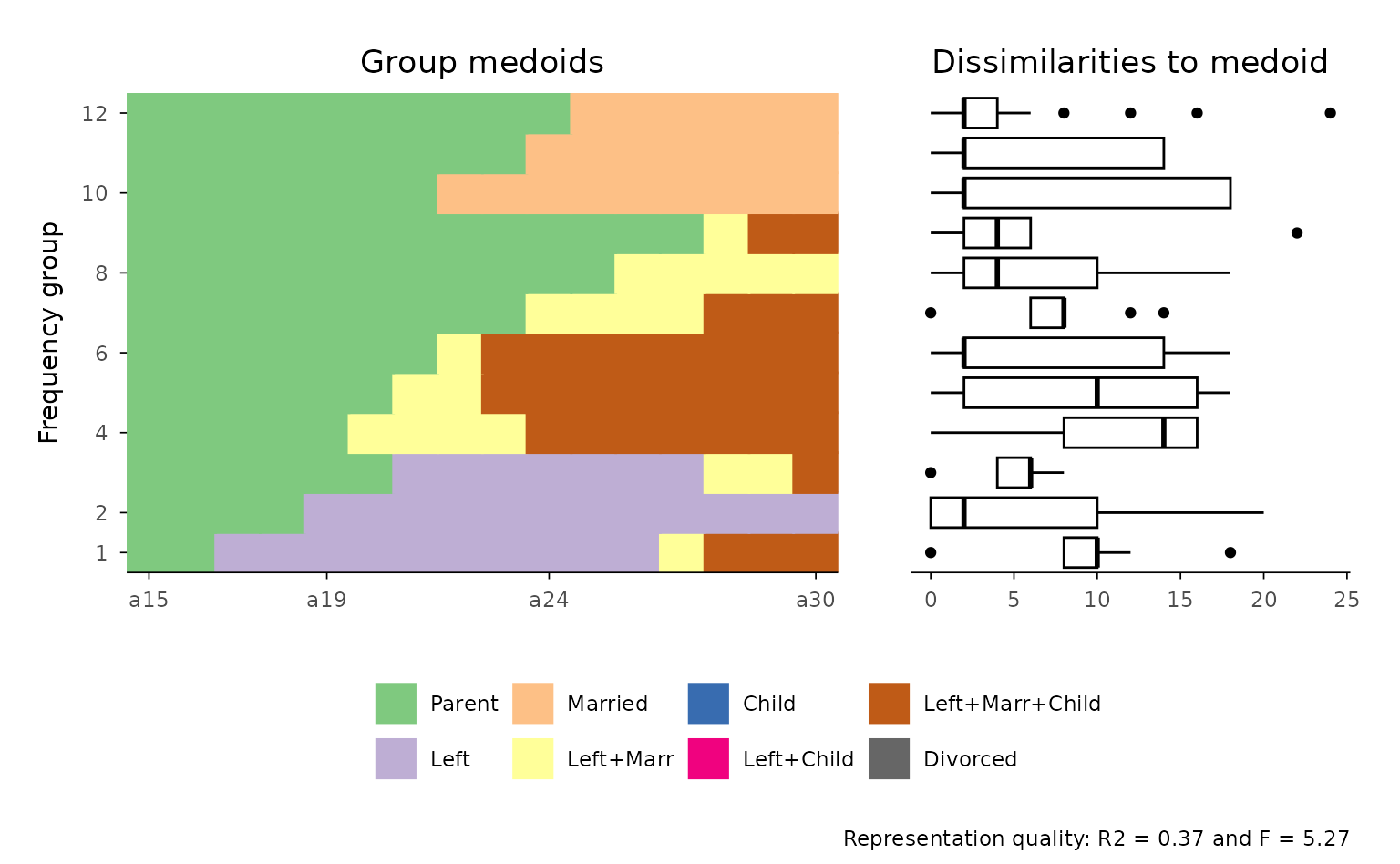p[] <- p[] +
scale_x_discrete(labels = month.abb)
#> Scale for x is already present.
#> Adding another scale for x, which will replace the existing scale.

p[] <- p[] +
scale_x_discrete(labels = month.abb)
#> Scale for x is already present.
#> Adding another scale for x, which will replace the existing scale.

p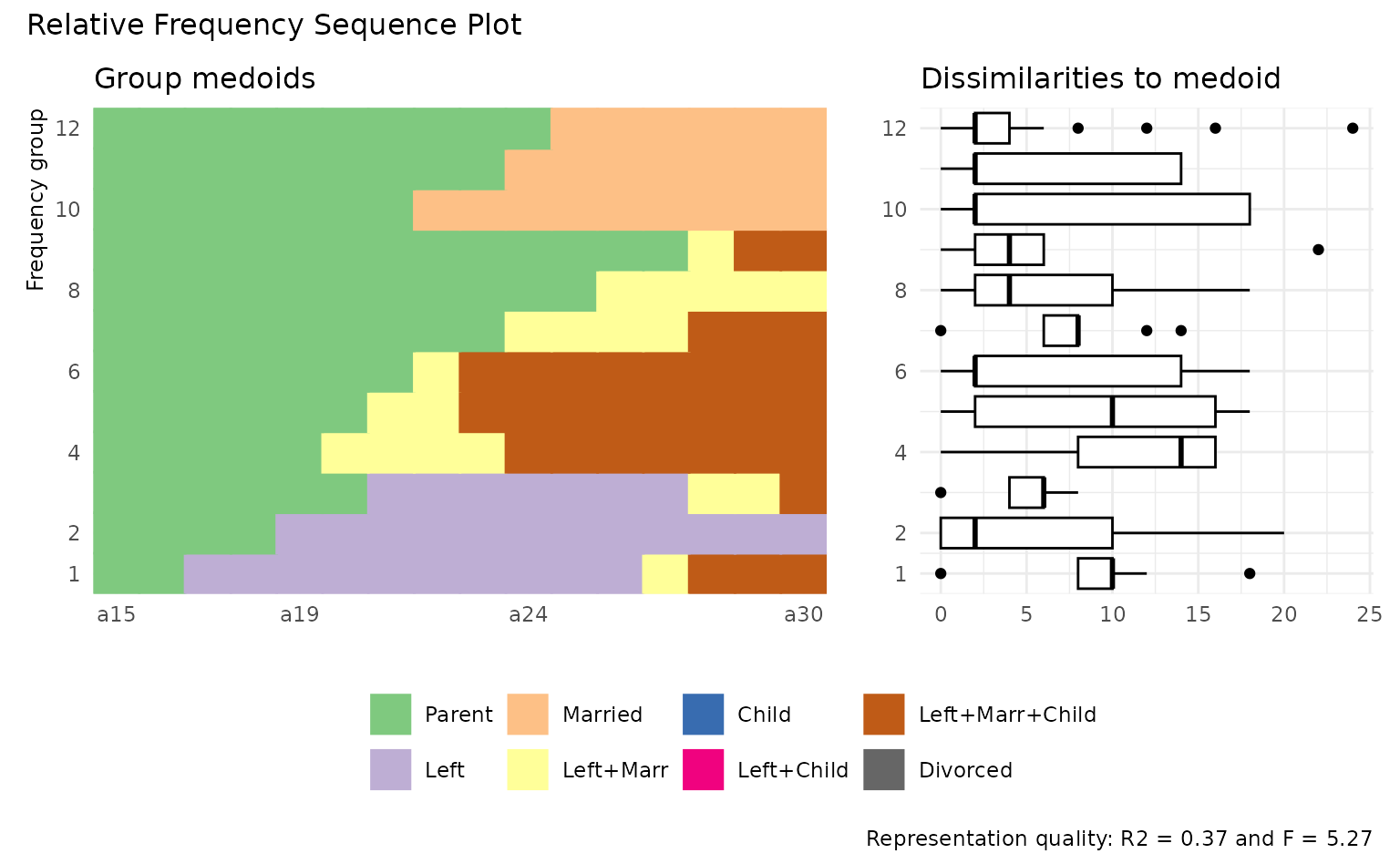#### Example 6: What about grouped rfplots?

Grouped rfplots are currently not implemented for ggseqrfplot and have to be created manually using the {patchwork} library.

In the following example we

1. create rfplots for our groups (here “Men” and “Women”), then proceed by
2. slightly misusing {patchwork}’s tag annotation assigning group-specific tags for the first plot in each row of the final patchwork plot,
3. removing the legend from the upper plot panel (pman), 4. and arranging the adjusted patches in a final plot with two stacked panels. Technically speaking the resulting plot is a nested patchwork plot. According to the documentation of {patchwork} [i]t is important to note that plot annotations only have an effect on the top-level patchwork. Any annotation added to nested patchworks are (currently) lost. If you need to have annotations for a nested patchwork you’ll need to wrap it in wrap_elements() with the side-effect that alignment no longer works.1 For this reason the group-specific titles were not added with patchwork::plot_annotation or ggplot2::ggtitle and we reverted to the use of tags instead.  diss <- seqdist(biofam.seq, method = "LCS") #> [>] 100 sequences with 8 distinct states #> [>] creating a 'sm' with a substitution cost of 2 #> [>] creating 8x8 substitution-cost matrix using 2 as constant value #> [>] 76 distinct sequences #> [>] min/max sequence lengths: 16/16 #> [>] computing distances using the LCS metric #> [>] elapsed time: 0.02 secs sex <- biofam[501:600, "sex"] p <- map2( levels(sex), # input x c("Men", "Women"), # input y function(x, y) { p <- ggseqrfplot(biofam.seq[sex == x,], diss = diss[sex == x,sex == x], k = 12) p[] <- p[] + labs(tag = y) return(p) } ) #> [>] Using k=12 frequency groups with grp.meth='prop' #> [>] Pseudo/medoid-based-R2: 0.5448432 #> [>] Pseudo/medoid-based-F statistic: 3.408139, p-value: 0.003407164 #> [>] Using k=12 frequency groups with grp.meth='prop' #> [>] Pseudo/medoid-based-R2: 0.5263291 #> [>] Pseudo/medoid-based-F statistic: 5.687386, p-value: 4.537004e-06 names(p) <- levels(sex) (pman & theme(legend.position = "none")) / p\$woman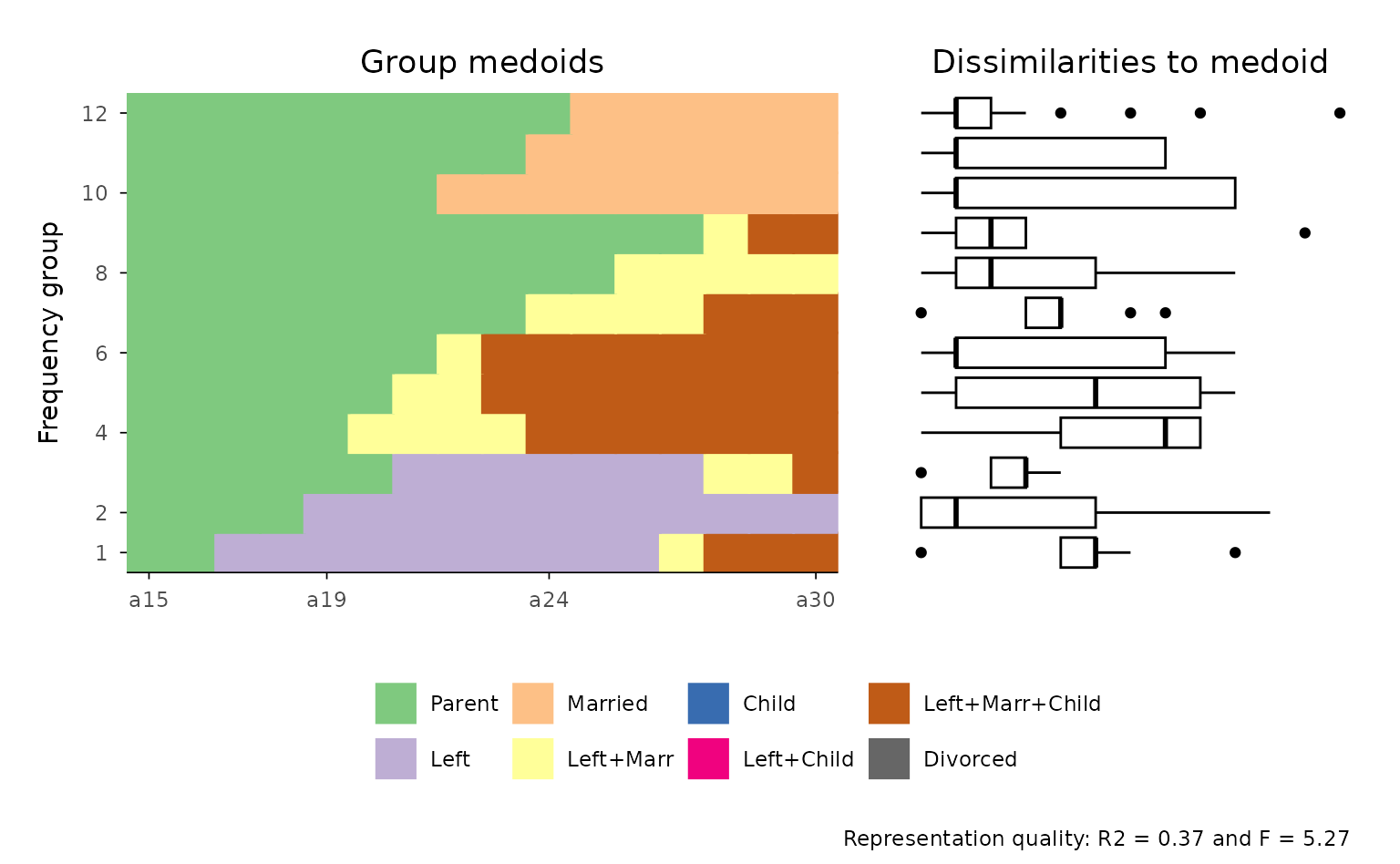Brzinsky-Fay, C. (2014). Graphical Representation of Transitions and Sequences (P. Blanchard, F. Bühlmann, & J.-A. Gauthier, Eds.; pp. 265–284). Springer. https://doi.org/10.1007/978-3-319-04969-4_14
Fasang, A. E., & Liao, T. F. (2014). Visualizing Sequences in the Social Sciences: Relative Frequency Sequence Plots. Sociological Methods & Research, 43(4), 643–676. https://doi.org/10.1177/0049124113506563
Gabadinho, A., Ritschard, G., Müller, N. S., & Studer, M. (2011). Analyzing and Visualizing State Sequences in R with TraMineR. Journal of Statistical Software, 40(4), 1–37. https://doi.org/10.18637/jss.v040.i04
Pedersen, T. L. (2020). Patchwork: The composer of plots. https://CRAN.R-project.org/package=patchwork
Piccarreta, R., & Lior, O. (2010). Exploring sequences: A graphical tool based on multi-dimensional scaling: Exploring Sequences. Journal of the Royal Statistical Society: Series A (Statistics in Society), 173(1), 165–184. https://doi.org/10.1111/j.1467-985X.2009.00606.x
Raab, M., & Struffolino, E. (2022). Sequence analysis (Vol. 190). SAGE. https://sa-book.github.io/
Wickham, H. (2016). ggplot2: Elegant graphics for data analysis (2nd ed. 2016). Springer. https://doi.org/10.1007/978-3-319-24277-4